• 1. 目标 编写一个支持浮点数及括号的加减乘除计算器。 输入：中缀表达式 输出：后缀表达式及计算结果 注意：该代码在VS13上运行通过。 运行示例： 2. 实现流程 2.1 中缀表达式转换为后缀表达式请参考如下...#

<span style="font-family: Arial, Helvetica, sans-serif; background-color: rgb(255, 255, 255);">1. 目标</span>

编写一个支持浮点数及括号的加减乘除计算器。
输入：中缀表达式
输出：后缀表达式及计算结果
注意：该代码在VS13上运行通过。
运行示例：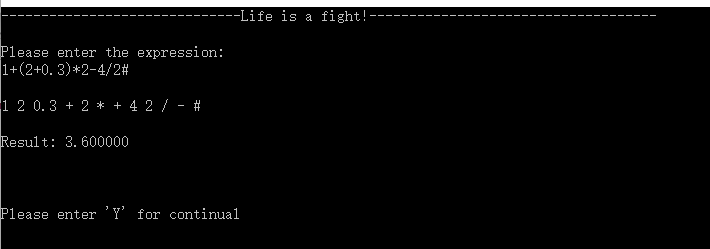2. 实现流程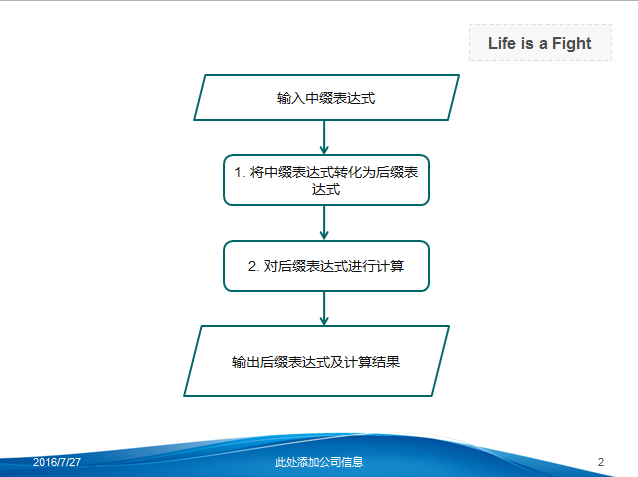2.1 中缀表达式转换为后缀表达式请参考如下链接：点击打开链接

3. 源代码

</pre><p><pre name="code" class="objc" style="font-size: 18px; font-weight: bold;">#include<stdio.h>
#include<ctype.h>
#include<stdlib.h>
#define stacksize 30
#define stackincrease 30
#define maxvalume 30
#define expvalume 200
#define title "------------------------------Life is a fight!------------------------------------"

typedef float Elemtype;

typedef struct STACK{
Elemtype* top;
Elemtype* base;
int len;
} stack;

stack* InitStack()
{
Elemtype* t;
stack* s;
t=(Elemtype*)malloc(stacksize*sizeof(Elemtype));
s=(stack*)malloc(sizeof(stack));
s->top=t;
s->base=t;
s->len=stacksize;
return s;
}

void PushStack(stack *s, Elemtype d)
{
if(s->top-s->base>=s->len-1)
s->base=(Elemtype*)realloc(s->base,(s->len+stackincrease)*sizeof(Elemtype));
*s->top=d;
s->top++;
}

void PopStack(stack* s, Elemtype* d)
{
if(s->top==s->base)
{
printf("\nErr!\n");
return;
}
*d=*--(s->top);
}

int LengthStack(stack *s)
{
return (s->top-s->base);
}

int GetExp(Elemtype* str)//读取输入的中缀表达式，保存到str数组中
{
char c;
int i=0;
fflush(stdin);
scanf("%c",&c);
while(c!='\n')
{
if(i>expvalume)
{
printf("Err: The expression is too long!\n");
return -1;
}
*str++=c;
i++;
scanf("%c",&c);
}
if(i==0)
{
*str++='#';
}
*str='\0';
return i;
}

void NiftoSuf(Elemtype* nif, Elemtype* suf)//将中缀表达式（nif）转换为后缀表达式(suf)
{
int i, j, n;
Elemtype c, t;
stack *s;

i=0;
j=0;
s=InitStack();
c=(char)nif[i];
while(c!='#')//'#'为输入结束标志
{
while(isdigit(c)||c=='.')//以字符的方式读取数字,将数字复制到suf中
{
suf[j++]=c;
c=(char)nif[++i];
if((c<'0'||c>'9')&&c!='.')
{
suf[j++]=' ';
}
suf[j]='\0';
}
if(c==')')
{
PopStack(s, &t);
while(t!='(')
{
suf[j++]=t;
suf[j++]=' ';
PopStack(s,&t);
}
suf[j]='\0';
}
else if(c=='(')
PushStack(s,c);
else if(c=='+'||c=='-')
{
if(!LengthStack(s))
{
PushStack(s,c);
}
else
{
do{
PopStack(s,&t);
if(t=='(')
{
PushStack(s,t);
}
else
{   suf[j++]=t;
suf[j++]=' ';
suf[j]='\0';
}
} while(LengthStack(s)&&t!='('&&c!='(');
PushStack(s,c);
}
}
else if(c=='*'||c=='/'||c=='(')
{
PushStack(s,c);
}
else if(c=='#' )
{
while(LengthStack(s))
{
PopStack(s,&t);
suf[j++]=t;
suf[j++]=' ';
}
break;
}
else
{
printf("\nErr:Expression Error\n");
return -1;
}
i++;
c=nif[i];
}
if(c=='#')
while(LengthStack(s))
{
PopStack(s,&t);
suf[j++]=t;
suf[j++]=' ';
}
suf[j++]='#';
suf[j]='\0';
free(s);
}

Elemtype Cal(Elemtype* suf)
{
int i, j;
char c;
Elemtype f, r, d1, d2;
stack *s;

i=0;
j=0;
s=InitStack();
char t[maxvalume];
c=suf[j++];
while(c!='#')
{
while(isdigit(c)||c=='.')
{
if(i>maxvalume)
{
printf("Err: The data is too large!\n");
return -1;
}
t[i++]=c;
t[i]='\0';
c=suf[j++];
if(c==' ')
{
f=atof(t);
PushStack(s,f);
i=0;
}
}
switch (c){
case '+':
PopStack(s,&d2);
PopStack(s,&d1);
PushStack(s,d1+d2);
break;
case '-':
PopStack(s,&d2);
PopStack(s,&d1);
PushStack(s,d1-d2);
break;
case '*':
PopStack(s,&d2);
PopStack(s,&d1);
PushStack(s,d1*d2);
break;
case '/':
PopStack(s,&d2);
if(d2==0)
{
printf("Err: data error!\n");
return  -1;
}
PopStack(s,&d1);
PushStack(s,d1/d2);
break;
}
c=suf[j++];
}
PopStack(s,&r);
printf("Result: %f",r);
free(s);
return r;
}

int main(void)
{
int i,j,k;
char c,c1,y;
Elemtype nif[expvalume], suf[expvalume];
printf("%s\n\n",title);

do{
i=0;
j=0;
k=0;
GetExp(nif);
NiftoSuf(nif,suf);
printf("\n");
while(suf[k]!='\0')
{
c1=suf[k];
printf("%c",c1);
k++;
}
printf("\n\n");
Cal(suf);
printf("\n\n\n");
scanf("%c",&y);
printf("\n");
fflush(stdin);
} while(y=='Y'||y=='y');
return 0;
}

展开全文• 的定义及存储 只能在固定一端进行插入和删除操作的线性结构。进行插入删除操作的一端称为栈顶，...顺序除了单个外还有共享，所谓共享就是两个共用一快储存空间，当然共享一般用于两个空间需求相反的
栈的定义及存储  只能在固定一端进行插入和删除操作的线性结构。进行插入删除操作的一端称为栈顶，另一端称为栈底，插入数据的操作称为压栈，删除数据的操作称为出栈。  栈的储存结构有顺序栈和链链两种。  顺序栈和顺序表基本一样，不同的是顺序栈只能固定在栈顶进行操作。顺序栈除了单个栈外还有共享栈，所谓共享栈就是两个栈共用一快储存空间，当然共享栈一般用于两个栈空间需求相反的情况下，比如一个增加一个减少，这就可以用共享栈了。  链栈可用单链表实现，一般将链表的头作为栈顶，链表的尾部作为栈底，这种情况下采用首插和首删法将比尾插和尾删更高效，因此在链栈中采用首插和首删法进行进栈和出栈的操作。  那么这两种储存方式哪种好一些呢？就进行时间复杂度比较而言两种方式都差不多，然而我们知道链表在内存中储存是碎片化的，所以比较浪费空间，因此就空间存储上顺序栈要优越一点。因此我们在这里实现一个顺序栈，并用实现的顺序栈做一个实例—–括号匹配。  顺序栈的实现  栈的基本操作有入栈、出栈、判空、取栈顶元素、计算栈内元素个数。下面我们用C++语言来实现：
template<typename T>
class Stack
{
public:
Stack()
:_array(NULL)
,_size(0)
, _capacity(0)
{}
~Stack()
{
if (_array)
{
delete[] _array;
}
}
void Push(const T& data)
{
CheckCapacity();
_array[_size++] = data;
}
void Pop()
{
if (!Empty())
{
_size--;
}
}
T& Top()
{
assert(_size != 0);
return _array[_size-1];
}
T& Top()const
{
assert(_size != 0)
return _array[_size-1];
}
size_t Size()const
{
return _size;
}
bool Empty()const
{
return !_size;
}
private:
void CheckCapacity()
{
if (_size == _capacity)
{
_capacity = _capacity * 2 + 3;
T *ptemp = new T[_capacity];
if (_size)
{
for (size_t i = 0; i < _size; i++)
{
ptemp[i] = _array[i];
}
delete[] _array;
}
_array = ptemp;
}
}

T* _array;
size_t _capacity;
size_t _size;
};
这里写了一个模板类，当用某个类型来对这个模板进行实例化后就可以对这个栈进行压栈和出栈操作了。下面我们来用用这个栈——括号匹配。  栈的简单运用之括号匹配  这里的括号以C++程序中常用的三种括号()、[]、{}为例，我们要用程序来判断一段字符串的括号匹配是否正确。  括号通常有四种情况：  （1）、左括号多了。  （2）、右括号多了。  （3）、括号顺序错了。  （4）、括号匹配正确。  下面给出实现代码：
bool MatchBrackets(char* pStr)
{
assert(pStr != NULL);
Stack<char> stack_char;
size_t len = strlen(pStr);
for (size_t i = 0; i < len; i++)
{
if (pStr[i] != '(' && pStr[i] != ')'&&
pStr[i] != '[' && pStr[i] != ']'&&
pStr[i] != '{' && pStr[i] != '}')
continue;
if (pStr[i] == '(' || pStr[i] == '[' || pStr[i] == '{')
{
stack_char.Push(pStr[i]);
}
else
{
if (stack_char.Empty())
{
cout << "右括号多了" << endl;
return false;
}
char ch = stack_char.Top();
if (ch == '(' && pStr[i] == ')' ||
ch == '['&&pStr[i] == ']' ||
ch == '{'&&pStr[i] == '}')
{
stack_char.Pop();
}
else
return false;
}
}
if (!stack_char.Empty())
{
cout << "左括号多了" << endl;
return false;
}
return true;
}
当括号匹配成功时就返回true，否则返回false，我们来看看测试：  先给出左括号多的例子：  void test1()  {  char str[] = “abc{cd(dfsfs[fksd]}”;  if (MatchBrackets(str))  cout << “匹配正确”;  }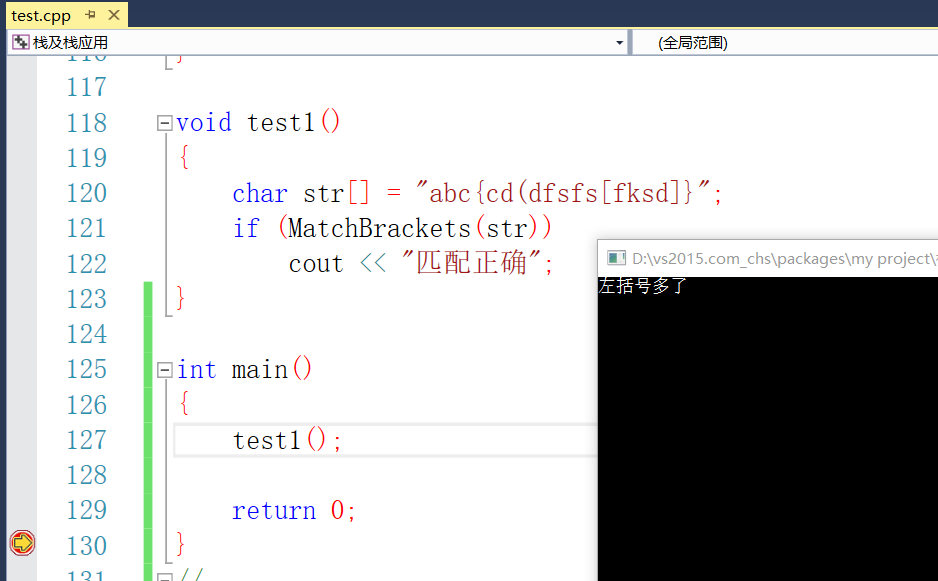再给出右括号多的情况：
void test2()
{
char str[] = "abc{cd(dfs)fs[fksd]}}";
if (MatchBrackets(str))
cout << "匹配正确";
}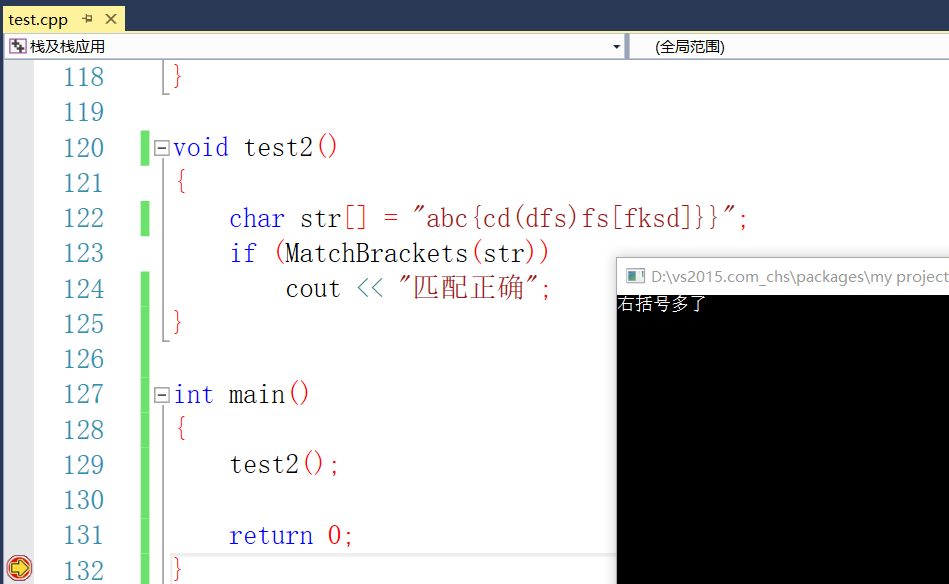匹配错误：
void test2()
{
char str[] = "abc{cd(dfs)f)s[fksd]}";
if (MatchBrackets(str))
cout << "匹配正确";
else
cout << "匹配错误";
}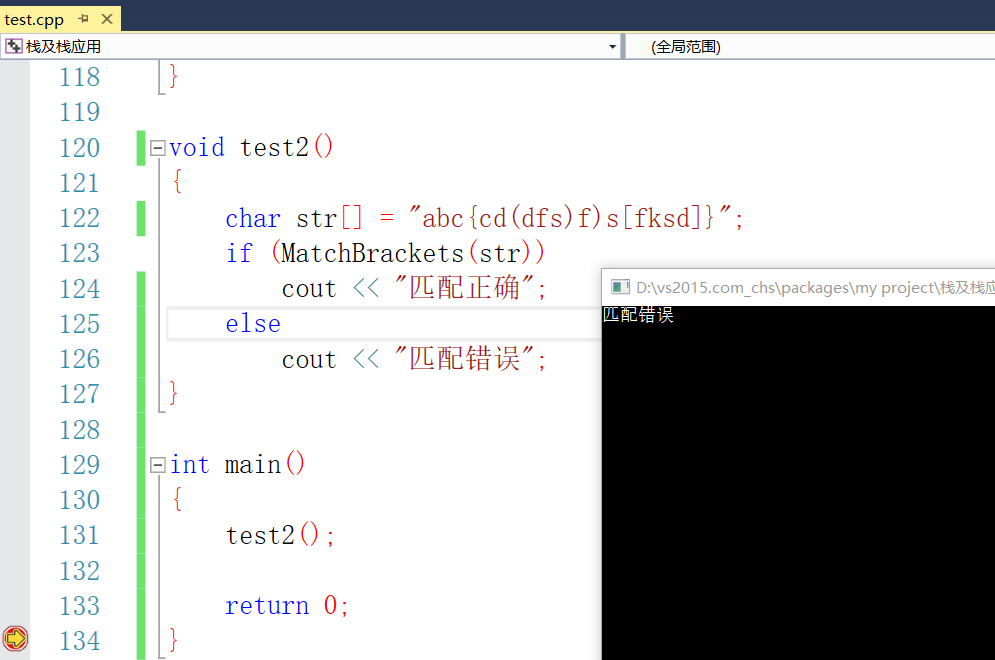正确匹配：
void test2()
{
char str[] = "abc{cd(dfs)fs[fksd]}";
if (MatchBrackets(str))
cout << "匹配正确";
else
cout << "匹配错误";
}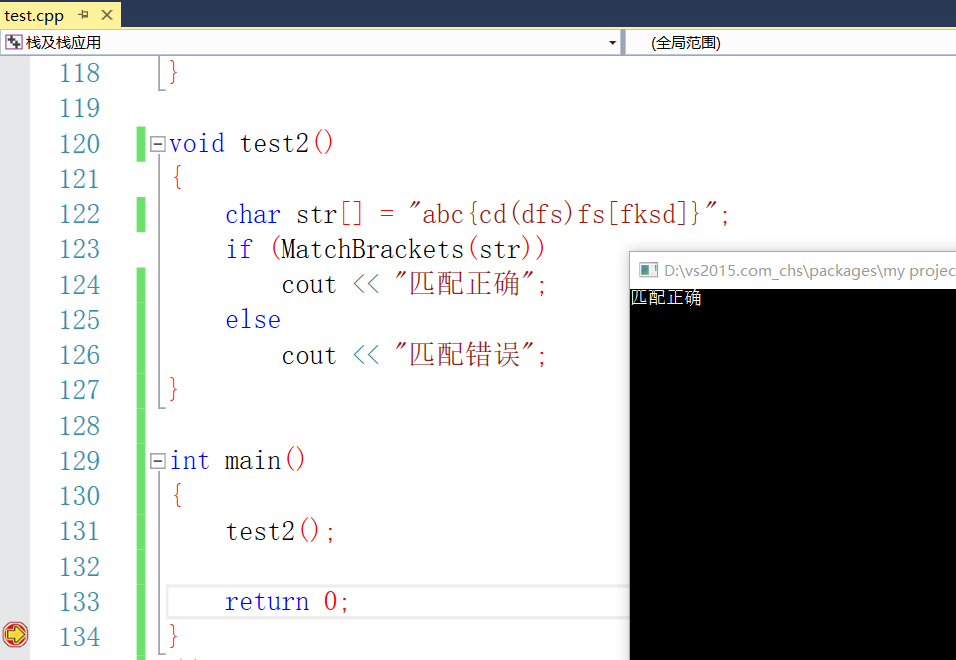以上就是栈的描述及栈的简单运用—括号匹配，在后面的文章会运用栈来进行更深一点的运用。欢迎各位勘误。
展开全文数据结构 括号匹配 C++
• 是计算机术语中比较重要的概念，实质上就是一段内存区域，但是满足一定的特性，那就是只有一个口，具有先入后出的特性，这种特性在计算机中有很广泛的运用。其实在程序员无时无刻不在运用栈，函数的调用是我们...
• 检验算法借助一个，每当读入一个左括号，则直接入栈，等待相匹配的同类右括号；每当读入一个右括号，若与当前栈顶的左括号类型相同，则二者匹配，将栈顶的左括号出栈，直到表达式扫描完毕在处理过程中，还要考虑...
1.案例分析
检验算法借助一个栈，每当读入一个左括号，则直接入栈，等待相匹配的同类右括号；每当读入一个右括号，若与当前栈顶的左括号类型相同，则二者匹配，将栈顶的左括号出栈，直到表达式扫描完毕在处理过程中，还要考虑括号不匹配出错的情况。
2.算法步骤
①初始化一个空栈S②设置一标记性变flag，用来标记匹配结果以控制循环及返回结果，1表示正确匹配，0表示错误匹配，fag初值为1。 ③扫描表达式，依次读入字符ch，如果表达式没有扫描完毕或flag非零，则循环执行以下操作:
若ch是左括号“[”或“("，则将其压入栈；若ch是右括号“)”，则根据当前栈顶元素的值分情况考虑：若栈非空且栈顶元素是“(”，则正确匹配，否则错误匹配，fag置为0；若ch是右括号“]”，则根据当前栈顶元素的值分情况考虑：若栈非空且栈顶元素是"[",则正确匹配，否则错误匹配，flag置为0。④退出循环后，如果栈空且fag值为1，则匹配成功，返回true,否则返回false
3.算法描述
bool Matching()
{
stack<char> s;  // 定义一个stack容器
char ch, temp;
int flag = 1;     // 标记匹配结果以控制循环及返回结果

cin >> ch; //读入第一个字符

while (ch != '#' && flag) //假设表达式以“#”结尾
{
switch (ch)
{
case '[':
case '(':      // 若是左括号，则将其压入栈
s.push(ch);
break;

case ')':  //若是“)”，则根据当前栈顶元素的值分情况考虑
if (!s.empty() && s.top() == '(')  // 若栈非空且栈顶元素是“(”，则正确匹配
{
temp = s.top();
s.pop();
}
else
flag = 0;    // 若栈空或栈顶元素不是“(”，则错误失败
break;

case ']': //若是“]”，则根据当前栈顶元素的值分情况考虑
if (!s.empty() && s.top() == '[') // 若栈非空且栈顶元素是“[”，则正确匹配
{
temp = s.top();
s.pop();
}
else
flag = 0;         //若栈空或栈顶元素不是“[”，则错误匹配
break;
} //switch
cin >> ch; //继续读入下一个字符
} //while
if (s.empty() && flag)
return true;         // 匹配成功
else
return false;      // 匹配失败
}
4.代码实现
main.cpp
#include <iostream>
#include <cstdlib>
#include <stack>

using namespace std;

bool Matching()
{
stack<char> s;  // 定义一个stack容器
char ch, temp;
int flag = 1;     // 标记匹配结果以控制循环及返回结果

cin >> ch; //读入第一个字符

while (ch != '#' && flag) //假设表达式以“#”结尾
{
switch (ch)
{
case '[':
case '(':      // 若是左括号，则将其压入栈
s.push(ch);
break;

case ')':  //若是“)”，则根据当前栈顶元素的值分情况考虑
if (!s.empty() && s.top() == '(')  // 若栈非空且栈顶元素是“(”，则正确匹配
{
temp = s.top();
s.pop();
}
else
flag = 0;    // 若栈空或栈顶元素不是“(”，则错误失败
break;

case ']': //若是“]”，则根据当前栈顶元素的值分情况考虑
if (!s.empty() && s.top() == '[') // 若栈非空且栈顶元素是“[”，则正确匹配
{
temp = s.top();
s.pop();
}
else
flag = 0;         //若栈空或栈顶元素不是“[”，则错误匹配
break;
} //switch
cin >> ch; //继续读入下一个字符
} //while
if (s.empty() && flag)
return true;         // 匹配成功
else
return false;      // 匹配失败
}

int main()
{

cout << "请输入待匹配的表达式，以“#”结束：" << endl;
//int flag = (int)Matching();
if (Matching())
cout << "括号匹配成功！" << endl;
else
cout << "括号匹配失败！" << endl;

system("pause");

return 0;
}
Leetcode 20题代码
class Solution {
public:
bool isValid(string s)
{
stack<char> S;  // 定义一个stack容器
char temp;
int flag = 1;
int i = 0;// 标记匹配结果以控制循环及返回结果

while (s[i] != '\0'&& flag)
{
switch (s[i])
{
case '[':
case '(':
case '{':          // 若是左括号，则将其压入栈
S.push(s[i]);
break;

case ')':  //若是“)”，则根据当前栈顶元素的值分情况考虑
if (!S.empty() && S.top() == '(')  // 若栈非空且栈顶元素是“(”，则正确匹配
{
temp = S.top();
S.pop();
}
else
flag = 0;    // 若栈空或栈顶元素不是“(”，则错误失败
break;

case ']': //若是“]”，则根据当前栈顶元素的值分情况考虑
if (!S.empty() && S.top() == '[') // 若栈非空且栈顶元素是“[”，则正确匹配
{
temp = S.top();
S.pop();
}
else
flag = 0;         //若栈空或栈顶元素不是“[”，则错误匹配
break;

case '}': //若是“]”，则根据当前栈顶元素的值分情况考虑
if (!S.empty() && S.top() == '{') // 若栈非空且栈顶元素是“{”，则正确匹配
{
temp = S.top();
S.pop();
}
else
flag = 0;         //若栈空或栈顶元素不是“[”，则错误匹配
break;

} //switch
i++;
} //while
if (S.empty() && flag)
return true;         // 匹配成功
else
return false;      // 匹配失败
}
};
运行结果展开全文括号匹配检验
• 实验目的：1、掌握的...2、 运用栈原理完成设计的内容。 完成数字十进制到八进制的转换。 输入示例： 请输入需转换的数的个数： 3 请输入需转换的数： 28，58，190 输出示例： 转换结果为： 1、 34 2、 72 3、 276
• 使用c++语言编写的运用栈和后缀表达式的简单计算器。
• 抓心挠肺呀，最后经过大牛的帮助下终于明白了cout 的原理 cout是从右到左入栈的，过程如下 push endl push 'b' call M.get() //打印0c push eax //push 0 push endl push 'a'...
一次对类成员的输出试验让我十分困惑，以下是源码：
#include<iostream> using namespace std; class m { public: m():num(0){} int get(){cout<<num<<'c'<<endl;return num;} int set(int c) { num=c; return num; } private: int num; }; int main() {    m M;
cout<<M.set(12)<<'a'<<endl<<M.get()<<'b'<<endl;
return 0; }
本应输出的结果为
12a
12c
12b
然而运行结果是抓心挠肺呀，最后经过大牛的帮助下终于明白了cout 栈的原理

cout是从右到左入栈的，过程如下

push endl
push 'b'
call M.get()            //打印0c
push eax    //push 0
push endl
push 'a'
call M.set(12)
push eax       //push 12

也就是遇到函数时执行函数，把返回值压入栈内，最后依次输出

﻿﻿

展开全文• 题：输入一个10进制整数，输出16进制。  转化16进制数，首先应该...这样我们很容易想到运用栈的特性先进后出来保存得到模，最后全部打印出来；下面使用顺序实现： #include #include #define N 20 typedef ilinux Linux LINUX 数据结构
• 抽象数据类型及其实现 Data-Structure-and-Algorithm-in-Python/Chapter-1/1-1-mission.py # -*- coding: utf-8 -*- '''请在Begin-End之间补充代码, 完成Stack类''' class Stack(): # 创建空列表实现 def __...列表 python 字符串 数据结构
• —是一种特殊的线性表 （stack）又名堆栈，它是一种运算受限的线性表。限定仅在表尾进行插入和删除操作的线性表。这一端被称为栈顶，相对地，把另一端称为底。向一个插入新元素又称作进栈、入栈或压栈，...
• 运用与实现的定义基本知识点的顺序结构及实现两共享空间的链式存储结构及实现的应用 的定义 （stack）是限定在表尾进行插入和删除操作的线性表。又称先进后出的线性表，简称LIFO结构。 ...
• stack（）在android 中的运用：自定义了一个Activity管理Activity
• Java中的 我们在学数据结构的时候，学完数组，链表，就开始学习了，知道它是后进先出的，以及一些使用场景。 但是在我们的Java中到底如何使用呢，我们如何记住它的方法，知道它的类结构呢，下面开始总结，有了图...
• 这是运用栈来进行的数值转换，理解很简单，是在老师的要求下自己做的，可以下载下来看看，应该有写帮助，是一个压缩包，解压可以直接运行。
• 使用React技术开发SPA.该项目把一些平时工作、学习中 遇到的react案例抽离成demo展现出来.
•文档
• 使用实现表达式求值 题目要求 读取一个多项式，使用进行多项式求值，并输出结果。 ##思路 1.使用两个，nums用于存储操作数，ops用于存储操作符 2.从左往右扫描，遇到操作数入栈nums 3.遇到操作符时，如果...字符串 数据结构 stack c++
• case '/': if (b == 0) { //除数为零两种情况，一是中恰有两个元素，取出中两个元素，不计算结果放入中，导致为空 flag = -1; //给出除数为零的信息 cout !" ;//二是，中多于两个元素，取出...
• Spring Boot 技术的实战类课程，课程共分为 3 大部分，前面两个部分为基础环境准备和相关概念介绍，第三个部分是 Spring Boot 项目实践开发。Spring Boot 介绍、前后端分离、API 规范等内容旨在让读者更加熟悉 ...java java面试题 java面试 java学习路线
• **（Stack）**又称堆栈，它是一种运算受限的线性表，其限制是仅允许在表的一端进行插入和删除运算。人们把此端称为栈顶，栈顶的第一个元素被称为栈顶元素，相对地，把另一端称为底。向一个插入新元素又称为...
•文档
• 当将一个十进制整数N转换为八进制数时，在计算过程中，把N与8求余得到的八进制数的各位依次进栈，计算完毕后将中的八进制数依次出栈输出，输出结果就是待求得的八进制数。 2.算法步骤 ①初始化一个空栈S。 ②当...
• 输入两个整数序列，第一个序列表示的压入顺序，请判断第二个序列是否可能为该的弹出顺序。假设压入栈的所有数字均不相等。例如序列1,2,3,4,5是某的压入顺序，序列4,5,3,2,1是该压栈序列对应的一个弹出序列，但......